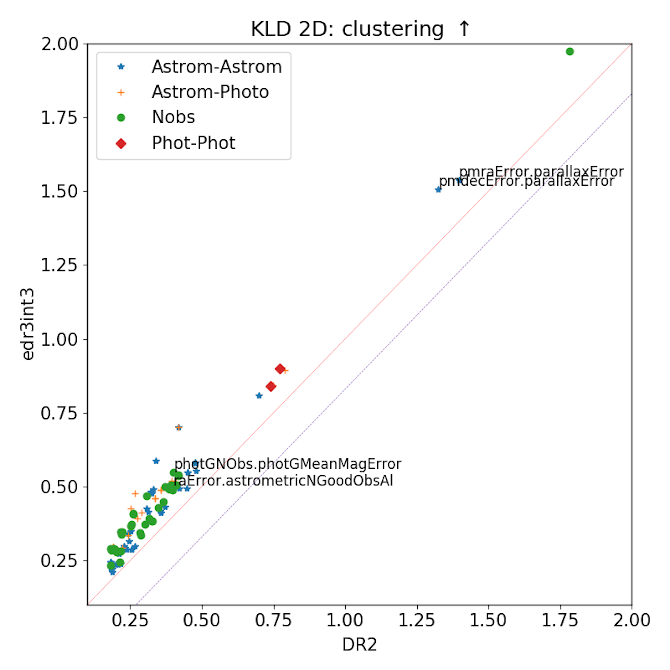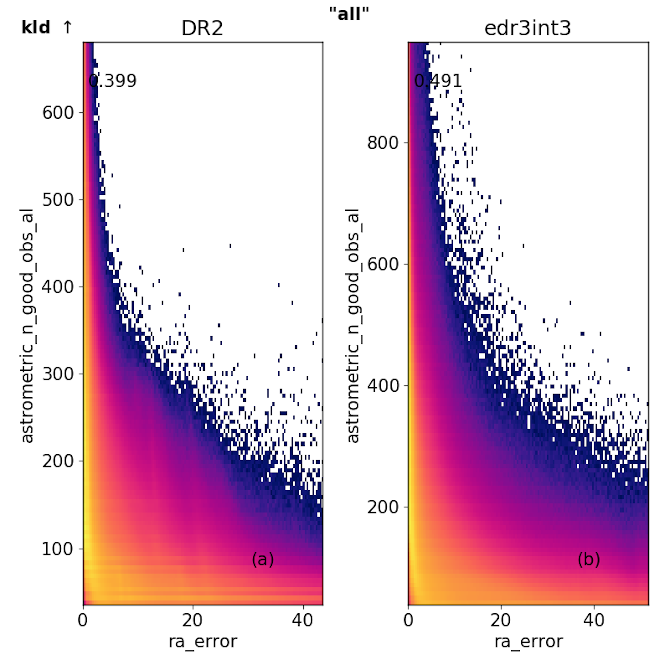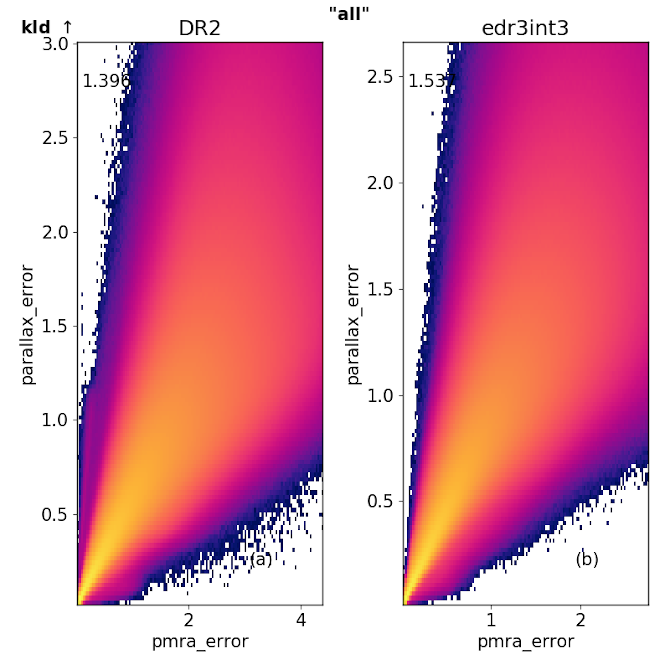8.5.3 Two dimensional KLD

The first test compares KLD values between edr3int3 and Gaia DR2 for all 2D subspaces. This is shown in Figure 8.20. In the left panel, we plot the 1:1 comparison between the KLD in the two datasets. The sets for which there is a deviation greater than 10% with respect to Gaia DR2 are coloured yellow (higher) and green (lower) respectively. We notice that while a few subspaces lie very close to the 1:1 line, there is a large set of subspaces for which the KLD has dropped (green circles) in edr3int3. Furthermore, these sets in green follow a parallel track to the 1:1 line, with an offset of about 0.17. Noticeably, there are very few subspaces for which the KLD has increased considerably.Figure 8.20: Comparison of two-dimensional KLD between edr3int3 and Gaia DR2. The 1:1 line is shown in red, while subspaces for which KLD has deviated by at least 10% with respect to Gaia DR2, are shown in yellow (increase), and green (decrease), respectively. The blue line is a guide to the low kld sequence at an offset of about 0.17 from the 1:1 line.Figure 8.21: Subspaces for which the two-dimensional KLD deviates by more than 10% in edr3int3 compared to Gaia DR2. Left panel: Subspaces for which the KLD has increased, and right panel: Subspaces for which the KLD has decreased. The colour-coding denotes subspace combinations of: Astrometry-Astrometry, Astrometry-Photometry, Photometry-Photometry, and those relating to Number of observations (Nobs). The labelled subspaces are those for which we choose to show histograms (Figure 8.22 etc.) as illustration.

For the subspaces where the KLD has increased, we notice that many of these contain astrometric uncertainties such as errors in the observables ra, dec, pmra, pmdec and parallax. In Figure 8.22 we show the 2D histograms for select subspaces where the KLD has increased in edr3int3. We see, for example, that The ra_error and dec_error have high KLD in combination with observables relating to the number of observations ($Nobs$). This is not surprising, and can be interpreted as a consequence of increased observations resulting in lower errors in astrometry. Another improvement is noted in the higher KLD between parallax_error & pmra_error, shown in Figure 8.23. In this case, the range of the errors in both proper motion and parallax has dropped i.e., they point towards improved astrometry in edr3int3.Figure 8.22: Example subspaces where the 2D KLD has increased in edr3int3 with respect to Gaia DR2. In this case, due to a stronger anti-correlation between number of observations and errors in astrometry and photometry.Figure 8.23: Example subspaces where the 2D KLD has increased in edr3int3 with respect to Gaia DR2. In this case, the range of errors in both proper motion and parallax has reduced, compared to Gaia DR2, resulting in higher clustering.

Conversely, in Figure 8.24, we show examples of where the KLD has dropped from Gaia DR2 to edr3int3. We note that the clustering between parallax_error and ra_error is lower. The range in both these errors is also smaller (especially for parallax_error). However, compared to Gaia DR2, the errors are less tightly correlated with each other, hence the lower clustering. In Gaia DR2, there are vertical striped patterns in the 2D distribution of this subspace, while in edr3int3, the distribution is smoother, and thus less clustered. Another example, is the comparison between parallax_over_error and phot_g_mean_flux_over_error. In this case, since the errors both in $parallax$ and $G$ magnitude, have dropped, the ranges for this subspace are much higher. As a result the KLD has dropped compared to Gaia DR2.Figure 8.24: Examples of subspaces where the 2D KLD has decreased in edr3int3 with respect to Gaia DR2.